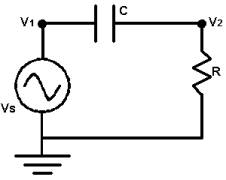Online JudgeProblem SetAuthorsOnline ContestsUser
Web Board
F.A.Qs
Statistical Charts
Problems
Submit Problem
Online Status
Prob.ID:
Register
Authors ranklist
Current Contest
Past Contests
Scheduled Contests
Award Contest
Register
Language:
Bode Plot
 Time Limit: 1000MS Memory Limit: 10000K Total Submissions: 17335 Accepted: 10434

Description

Consider the AC circuit below. We will assume that the circuit is in steady-state. Thus, the voltage at nodes 1 and 2 are given by v1 = VS coswt and v2 = VRcos (wt + q ) where VS is the voltage of the source, w is the frequency (in radians per second), and t is time. VR is the magnitude of the voltage drop across the resistor, and q is its phase.You are to write a program to determine VR for different values of w. You will need two laws of electricity to solve this problem. The first is Ohm's Law, which states v2 = iR where i is the current in the circuit, oriented clockwise. The second is i = C d/dt (v1-v2) which relates the current to the voltage on either side of the capacitor. "d/dt"indicates the derivative with respect to t.

Input

The input will consist of one or more lines. The first line contains three real numbers and a non-negative integer. The real numbers are VS, R, and C, in that order. The integer, n, is the number of test cases. The following n lines of the input will have one real number per line. Each of these numbers is the angular frequency, w.

Output

For each angular frequency in the input you are to output its corresponding VR on a single line. Each VR value output should be rounded to three digits after the decimal point.

Sample Input

```1.0 1.0 1.0 9
0.01
0.031623
0.1
0.31623
1.0
3.1623
10.0
31.623
100.0```

Sample Output

```0.010
0.032
0.100
0.302
0.707
0.953
0.995
1.000
1.000```

Source

[Submit]   [Go Back]   [Status]   [Discuss]Home PageGo BackTo top